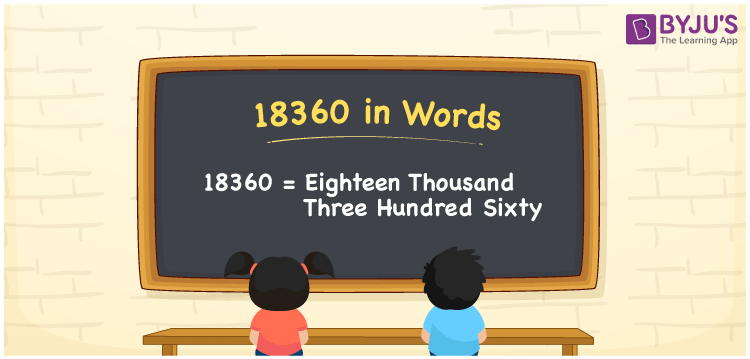# 18360 in Words

18360 in words is Eighteen Thousand Three Hundred Sixty. For example, if you spend Rs. 18360 in a month, you can write, “I have spent Rs. Eighteen Thousand Three Hundred Sixty in a month”. In general, the number name of any number can be written using the ones, tens, hundreds, and thousands place of a number. Thus, the place value chart is useful for writing the number 18360 in words.

 18360 in words Eighteen Thousand Three Hundred Sixty Eighteen Thousand Three Hundred Sixty in Numbers 18360

## 18360 in English Words## How to Write 18360 in Words?

We can convert 18360 to words using a place value chart. This can be done as follows. The number 18360 has 5 digits, so let’s make a chart that shows the place value up to 5 digits.

 Ten thousands Thousands Hundreds Tens Ones 1 8 3 6 0

Thus, we can write the expanded form as:

1 × Ten thousand + 8 × Thousand + 3 × Hundred + 6 × Ten + 0 × One

= 1 × 10000 + 8 × 1000 + 3 × 100 + 6 × 10 + 0 × 1

= 18360

= Eighteen Thousand Three Hundred Sixty

18360 is the natural number that is succeeded by 18359 and preceded by 18361.

18360 in words – Eighteen Thousand Three Hundred Sixty

Is 18360 an odd number? – No.

Is 18360 an even number? – Yes

Is 18360 a perfect square number? – No

Is 18360 a perfect cube number? – No

Is 18360 a prime number? – No

Is 18360 a composite number? – Yes

## Frequently Asked Questions on 18360 in Words

Q1

### How to write 18360 in words?

18360 in English is written as “Eighteen Thousand Three Hundred Sixty”.
Q2

### Is the number 18360 an odd number?

No, the number 18360 is not an odd number.
Q3

### Is 18360 a composite number?

Yes, 18360 is a composite number.